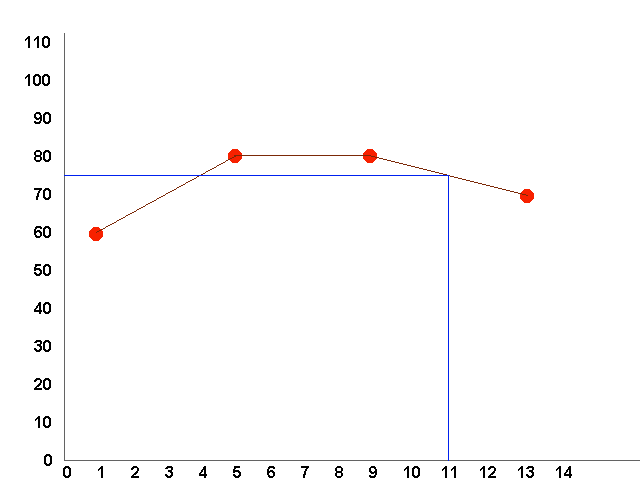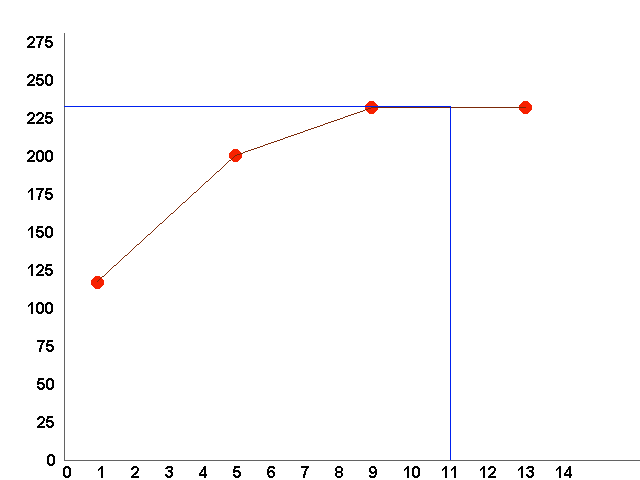Warning: include(/include/navi.inc): failed to open stream: No such file or directory in /kunden/chaospro.de/webseiten/anim.php on line 4

Warning: include(): Failed opening '/include/navi.inc' for inclusion (include_path='.:/usr/local/lib/php') in /kunden/chaospro.de/webseiten/anim.php on line 4

### Tutorial for Creating Animations

ChaosPro is able to automatically create sequences of fractal pictures. During this sequence any parameter (and any number of parameters) can be changed, just as the user wishes. The basic idea is as follows:

The user adds a fractal data structure (a data set which completely describes a fractal - FractInt users may think of a data structure as an entry in a *.par file) to the animation system. This data structure is copied and inserted into the list of keyframes.

1. After that the user changes some parameters in the original data structure (for example, number of iterations, the parameter c, the area values, the rotation angle or whatever) and adds the changed data set to the animation system again.

2. The user continues in doing so as many times as he/she likes.

3. The user provides the animation system with some other parameters like the size of the animation, the type (sequence of *.png files or an *.avi file) and specifies how many images should be calculated between two key frames.

4. The user starts the process: ChaosPro then subsequently creates fractal data structures, which interpolate the given keyframes, calculates the fractals described by the data structures, saves the image and continues with the next part of the sequence.

For example lets assume the user subsequently adds keyframes with the following values:

• bailout=60, maxiter=120, horizontal view angle=30 degree

• bailout=80, maxiter=200, horizontal view angle=45 degree

• bailout=80, maxiter=230, horizontal view angle=70 degree

• bailout=70, maxiter=230, horizontal view angle=70 degree, zoomed in one time by double clicking with the mouse into the mid of the window

Assume, that the user chooses linear interpolation and wants to calculate 4 frames from one keyframe to the next one.

ChaosPro then examines these 4 keyframes (AnimKeys). Let us assume, that ChaosPro now wants to set up frame number 11. The program now sets up a function for the bailout value (as it changes during the sequence, ChaosPro will notice this without further interaction from the user's side, of course). Below there is an image describing how ChaosPro works.

The unit on the horizontal axis is the frame number, the vertical axis has the parameter values, i.e. at first the bailout values.

ChaosPro now fills in the values it knows, i.e. frame 1 has bailout=60, frame 5 (4 images should be calculated between 2 keyframes) has 80, frame 9 has the value 80, too, and frame 13 has bailout=70 (see the red circles).The user choosed linear interpolation, so simply a polygon is drawn to connect the 4 points. ChaosPro then reads the value at point (image number) 11, gets 75, and this is how the bailout value for frame 11 is calculated.

Then the maximum number of iterations should be determined for frame number 11.

Again ChaosPro fills in the values known to the program, i.e. frame 1 has maxit=120, frame 5 has 200, frame 9 has the value 230 and frame 13 has maxit=230,too (see the red circles).As you can see, image 11 has the value 230, so the iteration value for the data structure, which will be created, when image 11 should be calculated, will be 230.

This method is applied to all parameters. ChaosPro examines the parameters, recognizes whether the parameter changes and interpolates it. The interpolation type specifies how the points are connected. 'Linear' simply draws lines between them (like above), 'Spline' calculates a cubic spline (i.e. a smooth curve which interpolates the given points). Most of the time you will use 'Spline' as interpolation type, as this generates smooth animations. The examples above use 'Linear' for simplicity.

So you can calculate an animation, where you look onto a 3D-transformation of a fractal (a kind of a landscape), then ' dive into' this fractal (you did some zooming on the 2D fractal), 'fly forward', (you moved the 2D fractal a bit around) while the palette changes and the fractal seems to morph (perhaps it was a juliaset and the user changed the parameter C of it, which gives some kind of morphing...) and at the end the light source moves to the back and vanishes...

I hope that you recognized the power of this algorithm: Inside ChaosPro a fractal has a specific data structure of a predefined format, all relations to other objects like formulas, palettes are defined. A parameter belongs either to the fractal and thus resides in the fractal data structure or it does not belong to the fractal and therefore is some global setting. This strict separation makes it possible to compare different fractal data structures and their parameters and to create animations of almost all parameters in a fractal.

Note: You can't change all parameters. Some of them act like switches (boolean values), and as you can imagine, there's no reasonable way to create sequences of images, if a switch changes. ChaosPro will notice you, if you try to create such a sequence of images as soon as you try to add your fractal data structure as an AnimKey.

## The way an animation normally gets created

Now let me explain how I create animations, and how you should create animations:

1. Calculate a fractal, which should define the starting point of the animation. Change it, so it suits your (optical) needs.

2. As soon as you are finished, open the animation window (Menu: Windows / Animation), choose the keyframes tab, add the fractal to the keyframes list by clicking onto the button Add First. This way a keyframe gets created and all values, which describe the fractal, are copied into this keyframe structure. The values in this keyframe stay fixed.

3. After that change some parameters of the calculated fractal (the original one), perhaps zoom in a bit, or move around or change the bailout value or do something else.

4. After that add it again (the same fractal, but now with other values...) to the keyframe list after the first one (select the first keyframe and click onto the button 'Add' to insert the new keyframe directly after the current selection). ChaosPro is able to recognize the parameters, which change between keyframes, and can create in-between data structures, which represent some fading from the parameter set of one keyframe to the parameter set of a second keyframe. It should be obvious, that one shouldn't change too much parameters too strong from one keyframe to the next, because the resulting fractal images will be too different and thus the sequence won't look like an animation: ChaosPro will still interpolate using its algorithm and this algorithm works in every case, but if you do too many changes then in order to get a smooth animation you would have to calculate hundreds of frames between the two keyframes. I personally zoom in about once (by a factor of two), then I add it again as a keyframe. If you want to change parameters by a large amount, then do this step by step, add several keyframes.

5. Continue changing parameters and adding the fractal to the end of the keyframe list until you think you are finished. You can add as many keyframes as you like, there is no limitation except the memory in your computer.

6. Well, this was the creation of the keyframes. After that you can define the width, height, number of frames to be calculated between two keyframes etc.

7. If you have done all you want, click onto 'Start' in the keyframe tab of the animation window and wait for the animation creation to finish (which can last a long time...).

Once upon a time somebody complained that creating animations didn't work like expected. He did the following:

He took two fractal data sets and added them as keyframes. He expected, that ChaosPro would produce some kind of morphing from one fractal to the other. Well, the 2 fractals were totally different: They were of the same kind, etc., but the one was a deep zoom at one location, the other one was an even deeper zoom at another location. He calculated 50 frames and wondered, why there were only black images. Well, if he would have specified about 17 000 000 frames to calculate, then he would have calculated an animation lasting 188 hours (at 25 frames per second) and he would have seen, that ChaosPro zoomed in a bit and then moved around to finally reach the goal, i.e. the second keyframe. The images were black, because ChaosPro moved over the big lake - the Mandelbrot set - which was black in that case.

What I wanted to say: Don't try adding 2 fractal data structures as keyframes, if you don't exactly know what you are doing. You won't crash ChaosPro (except if there is a bug), but you perhaps could get confused. Always pay attention, that two successing keyframes don't have too different values, so the interpolation makes sense and produces reasonable values.

Of course, you can add two totally different key frames. ChaosPro will interpolate in every case. And if you specify enough frames to calculate between the two key frames, you indeed will get a smoooth animation from one key frame to the other one...

Last update on Nov 26 2002.